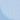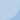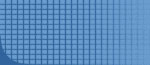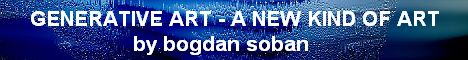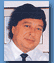Biography
 Home       ABOUT AUTHOR   biography   statement   curriculum   history   art critic GENERATIVE ARTGALERIESDEMO PROGRAMSOTHERCONTACT   contact KONEC LINKI GA konference  GA linki Drugi linki OBISKOVALCI ste obiskovalec  št.:  262988 od okt/2002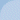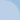DISCOVERING MANDELBROT 01 - program mandel03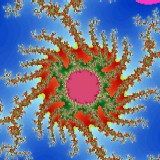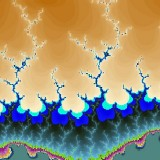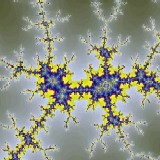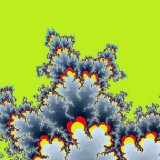Image properties: Picture box Picture1, Cartesian plain: x from –2 to +2; y from –2 to +2; dimension: width 500 pixels, height 500 pixels, command button for next image, click on image Result description: Basic concept: Algorithm description: Download and run exe version Visual Basic source code Public wid, hei, di, dj, zoomx1, zoomx2, zoomy1, zoomy2 Public fn1, fn2, fn3, f Private Sub Command1_Click()      Picture1.Cls: Call prepare: Call draw End Sub Private Sub Form_Load()      Form1.Show: Randomize Timer: Cls: wid = 500: hei = 500      Call prepare: Call draw End Sub Private Sub prepare()      di = 0: dj = 0: f = 10      fn1 = Rnd * 10: fn2 = Rnd * 10: fn3 = Rnd * 10      zoomx1 = Int(wid / 4): zoomx2 = 2: zoomy1 = Int(hei / 4): zoomy2 = 2 End Sub Private Sub draw()      On Error Resume Next      For i = 0 To wid: x = (i + di) / zoomx1 - zoomx2      For j = 0 To hei: y = zoomy2 - (j + dj) / zoomy1      zr = 0: zi = 0: zr2 = 0: zi2 = 0: cr = x: ci = y: n = 1      Do While n < 200 And zr2 + zi2 < 4           zr2 = zr * zr: zi2 = zi * zi           zi = 2 * zi * zr + ci: zr = zr2 - zi2 + cr: n = n + 1      Loop      re = (n * fn1) Mod 255: gr = (n * fn2) Mod 255: bl = (n * fn3) Mod 255      Picture1.PSet (i, j), RGB(re, gr, bl)      Next j: Next i      CurrentX = 55: CurrentY = 580: Print "Select interesting area and CLICK";: Print " *" End Sub Private Sub picture1_mousedown(button As Integer, shift As Integer, xt1 As Single, yt1 As Single)      xt = xt1: yt = yt1: di = di + xt - Int(wid / 2): dj = dj + yt - Int(hei / 2)      zoomx1 = zoomx1 * f: zoomx2 = zoomx2 * (1 / f)      zoomy1 = zoomy1 * f: zoomy2 = zoomy2 * (1 / f)      di = di * f: dj = dj * f: Cls: Call draw End Sub